## Permutations

### Learning Outcomes

• Use the addition principle to determine the total number of options for a given scenario.
• Use the multiplication principle to find the number of permutation of n distinct objects.
• Find the number of permutations of n distinct objects using a formula.
• Find the Number of Permutations of n Non-Distinct Objects.

## Using the Addition Principle

The company that sells customizable cases offers cases for tablets and smartphones. There are 3 supported tablet models and 5 supported smartphone models. The Addition Principle tells us that we can add the number of tablet options to the number of smartphone options to find the total number of options. By the Addition Principle there are 8 total options.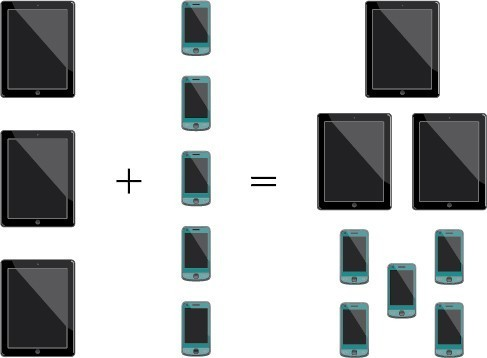### A General Note: The Addition Principle

According to the Addition Principle, if one event can occur in $m$ ways and a second event with no common outcomes can occur in $n$ ways, then the first or second event can occur in $m+n$ ways.

### Example: Using the Addition Principle

There are 2 vegetarian entrée options and 5 meat entrée options on a dinner menu. What is the total number of entrée options?

### Try It

A student is shopping for a new computer. He is deciding among 3 desktop computers and 4 laptop computers. What is the total number of computer options?

## Using the Multiplication Principle

The Multiplication Principle applies when we are making more than one selection. Suppose we are choosing an appetizer, an entrée, and a dessert. If there are 2 appetizer options, 3 entrée options, and 2 dessert options on a fixed-price dinner menu, there are a total of 12 possible choices of one each as shown in the tree diagram.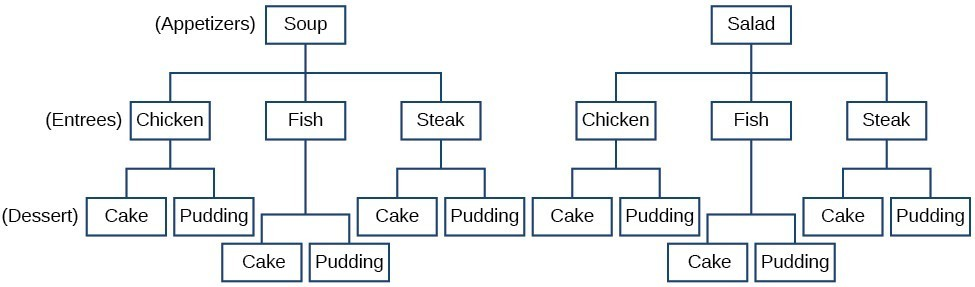The possible choices are:

1. soup, chicken, cake
2. soup, chicken, pudding
3. soup, fish, cake
4. soup, fish, pudding
5. soup, steak, cake
6. soup, steak, pudding
7. salad, chicken, cake
8. salad, chicken, pudding
9. salad, fish, cake
10. salad, fish, pudding
11. salad, steak, cake
12. salad, steak, pudding

We can also find the total number of possible dinners by multiplying.

We could also conclude that there are 12 possible dinner choices simply by applying the Multiplication Principle.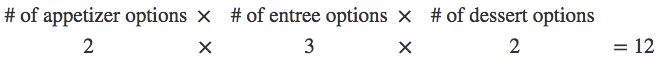### A General Note: The Multiplication Principle

According to the Multiplication Principle, if one event can occur in $m$ ways and a second event can occur in $n$ ways after the first event has occurred, then the two events can occur in $m\times n$ ways. This is also known as the Fundamental Counting Principle.

### Example: Using the Multiplication Principle

Diane packed 2 skirts, 4 blouses, and a sweater for her business trip. She will need to choose a skirt and a blouse for each outfit and decide whether to wear the sweater. Use the Multiplication Principle to find the total number of possible outfits.

### Try It

A restaurant offers a breakfast special that includes a breakfast sandwich, a side dish, and a beverage. There are 3 types of breakfast sandwiches, 4 side dish options, and 5 beverage choices. Find the total number of possible breakfast specials.

The Multiplication Principle can be used to solve a variety of problem types. One type of problem involves placing objects in order. We arrange letters into words and digits into numbers, line up for photographs, decorate rooms, and more. An ordering of objects is called a permutation.

## Finding the Number of Permutations of n Distinct Objects Using the Multiplication Principle

To solve permutation problems, it is often helpful to draw line segments for each option. That enables us to determine the number of each option so we can multiply. For instance, suppose we have four paintings, and we want to find the number of ways we can hang three of the paintings in order on the wall. We can draw three lines to represent the three places on the wall.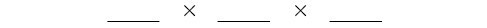There are four options for the first place, so we write a 4 on the first line.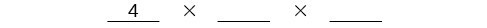After the first place has been filled, there are three options for the second place so we write a 3 on the second line.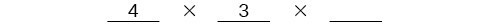After the second place has been filled, there are two options for the third place so we write a 2 on the third line. Finally, we find the product.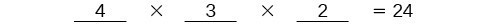There are 24 possible permutations of the paintings.

### How To: Given $n$ distinct options, determine how many permutations there are.

1. Determine how many options there are for the first situation.
2. Determine how many options are left for the second situation.
3. Continue until all of the spots are filled.
4. Multiply the numbers together.

### Example: Finding the Number of Permutations Using the Multiplication Principle

At a swimming competition, nine swimmers compete in a race.

1. How many ways can they place first, second, and third?
2. How many ways can they place first, second, and third if a swimmer named Ariel wins first place? (Assume there is only one contestant named Ariel.)
3. How many ways can all nine swimmers line up for a photo?

### Try It

A family of five is having portraits taken. Use the Multiplication Principle to find the following.
How many ways can the family line up for the portrait?

How many ways can the photographer line up 3 family members?

How many ways can the family line up for the portrait if the parents are required to stand on each end?

## Finding the Number of Permutations of n Distinct Objects Using a Formula

For some permutation problems, it is inconvenient to use the Multiplication Principle because there are so many numbers to multiply. Fortunately, we can solve these problems using a formula. Before we learn the formula, let’s look at two common notations for permutations. If we have a set of $n$ objects and we want to choose $r$ objects from the set in order, we write $P\left(n,r\right)$. Another way to write this is ${}_{n}{P}_{r}$, a notation commonly seen on computers and calculators. To calculate $P\left(n,r\right)$, we begin by finding $n!$, the number of ways to line up all $n$ objects. We then divide by $\left(n-r\right)!$ to cancel out the $\left(n-r\right)$ items that we do not wish to line up.

Let’s see how this works with a simple example. Imagine a club of six people. They need to elect a president, a vice president, and a treasurer. Six people can be elected president, any one of the five remaining people can be elected vice president, and any of the remaining four people could be elected treasurer. The number of ways this may be done is $6\times 5\times 4=120$. Using factorials, we get the same result.

$\dfrac{6!}{3!}=\dfrac{6\cdot 5\cdot 4\cdot 3!}{3!}=6\cdot 5\cdot 4=120$

There are 120 ways to select 3 officers in order from a club with 6 members. We refer to this as a permutation of 6 taken 3 at a time. The general formula is as follows.

$P\left(n,r\right)=\dfrac{n!}{\left(n-r\right)!}$

Note that the formula stills works if we are choosing all $n$ objects and placing them in order. In that case we would be dividing by $\left(n-n\right)!$ or $0!$, which we said earlier is equal to 1. So the number of permutations of $n$ objects taken $n$ at a time is $\frac{n!}{1}$ or just $n!\text{.}$

### A General Note: Formula for Permutations of n Distinct Objects

Given $n$ distinct objects, the number of ways to select $r$ objects from the set in order is

$P\left(n,r\right)=\dfrac{n!}{\left(n-r\right)!}$

### How To: Given a word problem, evaluate the possible permutations.

1. Identify $n$ from the given information.
2. Identify $r$ from the given information.
3. Replace $n$ and $r$ in the formula with the given values.
4. Evaluate.

### Example: Finding the Number of Permutations Using the Formula

A professor is creating an exam of 9 questions from a test bank of 12 questions. How many ways can she select and arrange the questions?

### Q & A

#### Could we have solved  using the Multiplication Principle?

Yes. We could have multiplied $15\cdot 14\cdot 13\cdot 12\cdot 11\cdot 10\cdot 9\cdot 8\cdot 7\cdot 6\cdot 5\cdot 4$ to find the same answer.

### Try It

A play has a cast of 7 actors preparing to make their curtain call. Use the permutation formula to find the following.

How many ways can the 7 actors line up?

How many ways can 5 of the 7 actors be chosen to line up?

## Finding the Number of Permutations of n Non-Distinct Objects

We have studied permutations where all of the objects involved were distinct. What happens if some of the objects are indistinguishable? For example, suppose there is a sheet of 12 stickers. If all of the stickers were distinct, there would be $12!$ ways to order the stickers. However, 4 of the stickers are identical stars, and 3 are identical moons. Because all of the objects are not distinct, many of the $12!$ permutations we counted are duplicates. The general formula for this situation is as follows.

$\dfrac{n!}{{r}_{1}!{r}_{2}!\dots {r}_{k}!}$

In this example, we need to divide by the number of ways to order the 4 stars and the ways to order the 3 moons to find the number of unique permutations of the stickers. There are $4!$ ways to order the stars and $3!$ ways to order the moon.

$\dfrac{12!}{4!3!}=3\text{,}326\text{,}400$

There are 3,326,400 ways to order the sheet of stickers.

### A General Note: Formula for Finding the Number of Permutations of n Non-Distinct Objects

If there are $n$ elements in a set and ${r}_{1}$ are alike, ${r}_{2}$ are alike, ${r}_{3}$ are alike, and so on through ${r}_{k}$, the number of permutations can be found by

$\dfrac{n!}{{r}_{1}!{r}_{2}!\dots {r}_{k}!}$

### Example: Finding the Number of Permutations of n Non-Distinct Objects

Find the number of rearrangements of the letters in the word DISTINCT.

### Try It

Find the number of rearrangements of the letters in the word CARRIER.

## Contribute!

Did you have an idea for improving this content? We’d love your input.